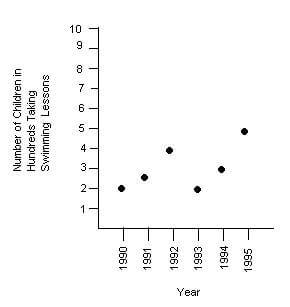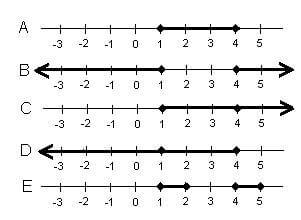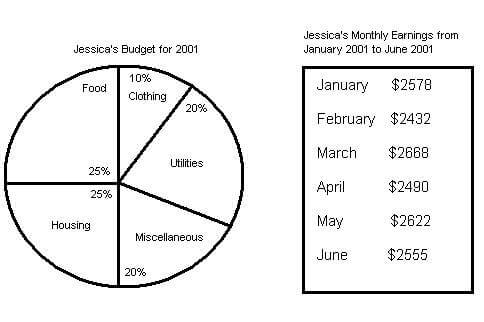# Mathematics Practice Questions 1

1. 325
2. 375
3. 400
4. 408
5. 425
1. y-z
2. z/y + 1
3. y(z-1)
4. z(y-1)
5. 1-zy
##### 3. Which of the following values is NOT equal to 34(58+9)?
1. 34 * 67
2. 58(34+9)
3. 34 * 58 + 34 * 9
4. 1,972 + 306
5. (9 + 58) 34
1. 50°
2. 55°
3. 60°
4. 80°
5. 90°
1. 863
2. 878
3. 896
4. 915
5. 932
##### 6. Which year did the most children take swimming lessons?1. 1990
2. 1991
3. 1992
4. 1994
5. 1995
1. 1990-1991
2. 1991-1992
3. 1992-1993
4. 1993-1994
5. 1994-1995
1. 250
2. 308
3. 385
4. 450
5. 1850
1. 0.0593
2. 0.00593
3. 593
4. 5930
5. 59300
1. 65 miles
2. 84 miles
3. 124 miles
4. 138 miles
5. 145 miles
##### 11. Which of the following is a correct graph of x>1, x<4 ?1. Line A
2. Line B
3. Line C
4. Line D
5. Line E
1. 18
2. 24
3. 32
4. 36
5. 43
1. 10
2. 15
3. 20
4. 25
5. 30
1. 20/30
2. 15/24
3. 25/30
4. 40/54
5. 2/7
1. 224.51
2. 237.6
3. 246.55
4. 271.38
5. 282.32
1. \$6,000
2. \$8,500
3. \$12,000
4. \$15,000
5. \$18,500
1. 50 feet
2. 75 feet
3. 120 feet
4. 130 feet
5. 150 feet
1. 8.1
2. 8.3
3. 8.6
4. 8.9
5. 9.0
1. \$32.48
2. \$64.96
3. \$97.44
4. \$129.92
5. \$194.88
1. 5
2. 10
3. 11
4. 12
5. 13
1. 10,000
2. 1,000
3. 0.1
4. 0.01
5. 0.001
1. 1
2. 2
3. 3
4. 4
5. 5
1. 1/9
2. 0
3. 1
4. 2
5. 3
1. 3
2. 7
3. 8
4. 9
5. 11
1. 1/20
2. 1/40
3. 1/50
4. 1/400
5. 1/500
1. 36
2. 37
3. 38
4. 39
5. 40
##### 27. How much money did Jessica’s budget allow for housing in April of 2001?1. \$617.80
2. \$620.92
3. \$622.50
4. \$626.38
5. \$633.20
1. \$249.90
2. \$250.40
3. \$251.32
4. \$253.33
5. \$255.75
1. \$131.10
2. \$144.30
3. \$148.32
4. \$152.22
5. \$153.33
1. 5 minutes
2. 10 minutes
3. 15 minutes
4. 18 minutes
5. 20 minutes
1. 7/8
2. 5/6
3. 3/4
4. 3/5
5. 5/7
1. 6/8
2. 8/6
3. 3/7
4. 8/14
5. 6/7
1. \$375
2. \$450
3. \$480
4. \$510
5. \$525
1. 16 feet
2. 35 feet
3. 37 feet
4. 40 feet
5. 42 feet
1. \$17,250.00
2. \$16,540.44
3. \$16,230.34
4. \$15,980.55
5. \$15,870.88
1. 3/2000
2. 2/750
3. 7/4000
4. 5/3462
5. 1/500
1. 0.0006334
2. 0.06334
3. 6334
4. 63340
5. 633400
1. -2
2. -1
3. 0
4. 1
5. 2
1. 60°
2. 70°
3. 80°
4. 90°
5. 120°
##### 40. Which of the following would be an appropriate unit to measure sugar for a cookie recipe?
1. liters
2. cups
3. quarts
4. kilograms
5. pounds

1. C: The equation, x-0.10x-0.15(x-0.10x)=306, may be used to solve the problem. Solving for x gives 0.90x – 0.15x + 0.015x = 306, where x = 400. Thus, the original price was \$400.

2. B: The equation may be solved by first distributing the y across the expression, x – 1, on the left side of the equation. Doing so gives: xy – y = Z. Adding y to both sides of the equation gives: xy = Z + y. Finally, division of both sides of the equation by y gives: x=(Z+y)/y or x=Z/y+1.

3. B: This problem illustrates the distributive property of multiplication over addition. The factor being distributed may not change.

4. D: The measure of the third angle of the triangle is equal to 180°-(15° + 85°), or 80°.

5. C: Since there are 32 ounces in 2 pounds (16 ounces = 1 pound), the following proportion may be written: 5/140=32/x . Solving for x gives x = 896. Thus, there are 896 grams in 2 pounds of meat.

6. E: The largest number of children taking swimming lessons, in one year, was 500, in 1995.

7. C: The only decrease in number of children taking swimming lessons was from 1992 to 1993, with a decrease of 200 children.

8. B: The average may be written as (200+250+400+200+300+500)/6, which is approximately 308.

9. A: Movement of the decimal point two places to the left gives 0.0593.

10. C: The following proportion may be used to solve the problem: 1/20=6.2/x. Solving for x gives x = 124, so there are actually 124 miles between the two towns.

11. A: The correct graph should show a line segment between 1 and 4, including the points, 1 and 4.

12. D: The volume of the tin is 972 in”. The volume of each piece of fudge is 27 in”. 972 ÷ 27 = 36.

13. B: The following system of equations may be used to solve the problem: (s=2b@sb=15). Substituting 2b for s, in the second equation, gives: 2b – b = 15, where b = 15. The younger brother is 15 years old.

14. C: Multiplying the numerator and denominator of the given fraction by 5 gives the fraction, 25/30, which is equivalent.

15. B: Converting feet to yards, the dimensions may be rewritten as 4 yards by 6 2/3 yards. Thus, the area of the floor is 26 2/3 yd’. Multiplication of this area by the cost per square yard gives the expression, 26 2/3?8.91, which equals 237.6. Thus, the cost is \$237.60.

16. C: The following equation may be solved for x: 6000=1/2 x. Solving for x gives x = 12,000. Thus, the amount of prize money distributed equaled \$12,000.

17. D: The distance may be determined by writing and solving the following equation for c: 502+1202=c2c = 130, thus the distance is 130 feet.

18. D: The average rate for the round trip is the total distance traveled divided by the total travel time. Distance traveled=2x. Travel time=x/10+x/8=4x/40+5x/40=9x/40. Average Rate=2xx9x/40=(2xx40)/9x=80/9 = approximately 8.9 mph.

19. E: The length is equal to 216 inches. The width is equal to 36 inches. So, the length may be covered by 18 12-inch stones, while the width may be covered by 3 12-inch stones. A total of 42 stones is needed for one layer, and 84 stones need for two layers. Multiplication of 84 by \$2.32 gives 194.88. Thus, the total cost is \$194.88.

20. E: The equation may be solved for x by first subtracting 6x from both sides of the equation. Doing so gives -3x = -15, where x = 5. Substituting 5 for x into the second expression gives 5 + 8, which equals 13.

21. B: There are 1,000 milliliters in 1 liter.

22. D: The problem may be modeled with the equation, 6.50 = 5.00 + 0.50x, where x represents the number of passengers. Solving for x gives x = 3. Thus, there were 3 passengers, plus 1 driver, for a total of 4 people in the vehicle.

23. C: This problem may be represented as 1/9.9, which equals 1.

24. D: Taking out 3 of each color will ensure that he has 1 of each color. Thus, he needs to take out 9 marbles, in all.

25. E: 0.20% = 0.002, and 1/500=0.002.

26. D: The increase from term to term is twice the increase from the previous two terms. Thus, the increase from 19 to the missing term will be 20, or twice the increase of 10. Thus, the missing term is equal to 19 + 20, or 39.

27. C: The solution may be modeled by the expression, 0.25(2490). Thus, her budget allowed \$622.50 for housing in April of 2001.

28. E: The average may be represented as (0.10(2578)+0.10(2432)+0.10(2668)+0.10(2490)+0.10(2622)+0.10(2555))/6, which simplifies as (257.80+243.20+266.80+249.00+262.20+255.50)/6, or 255.75. The average budget amount for clothing in the first six months of 2001 was \$255.75.

29. A: The amount she saved may be represented by the expression, 0.25(2622) – 0.20(2622), which equals 131.10. Thus, she saved \$131.10.

30. B: The problem may be modeled with the equation, 1/40+1/30+1/24=1/t. Solving for t gives t = 10. Thus, working together, they can type the same document in 10 minutes.

31. D: The fraction, 3/5, equals 0.6, which is less than 2/3.

32. C: The ratio may be written as 6/14, which reduces to 3/7.

33. B: The amount of money she made may be represented by the expression, 90 + 0.12x, where x represents the amount of sales. Substituting 3000 for x gives 90 + 0.12(3000), which equals 450. So, she made \$450 this week.

34. A: First, the perimeter measurement may be converted to feet. Multiplying 25 1/3 yards by 3 gives an equivalent measurement of 76 feet. Thus, the following equation may be written: 76 = 2(22) + 2w, which simplifies to 76 = 44 + 2w, where w = 16. The width of the house is 16 ft.

35. A: The problem may be modeled with the expression, 15,000 + 0.15(15,000), which equals 17,250. Thus, he sold the boat for \$17,250.

36. A: 3/2000=0.0015, which is equivalent to 0.15%.

37. D: Moving the decimal point 4 places to the right gives 63,340.

38. C: Solving the given equation for x gives x = -1. Substitution of -1 for x, in the second equation, gives -1 + 1 = 0.

39. A: The interior angle measures of a triangle sum to 180°. Thus, the measure of the third angle is equal to the difference of 180° and 120°, or 60°.

40. B: Cups is an appropriate measure of capacity for sugar.